All You Need to Know About

# Other Formulas in Excel

The Excel Other Formulas are not very well-known, but you will be surprised on the usefulness of these. We have in store for you Financial, Array Excel Formulas, and a lot of hidden nuggets in the Excel Formula world!

Here are the top things on what you can do with Other Formulas in Excel:

## Jump To A Cell Reference Within An Excel Formula

When writing, editing or auditing Excel formulas you will come across a scenario where you want to view and access the referenced cells within a formula argument.

This is helpful if you want to check how the formula works or to make any changes to the formula.

There is a cool tip where you can jump to the referenced cell or range within the formula and make your changes.

STEP 1: Double click inside your Excel formulaSTEP 2: Select the formula argument that you want to edit with your mouseSTEP 3: Press F5 which will bring up the Go To dialogue box and press OK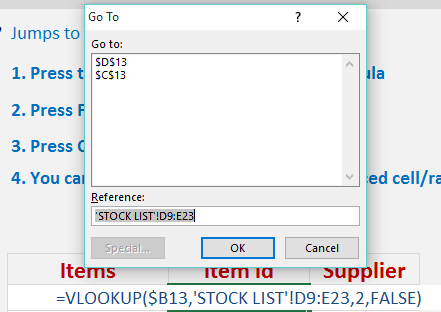STEP 4: This will take you to the referenced cell/range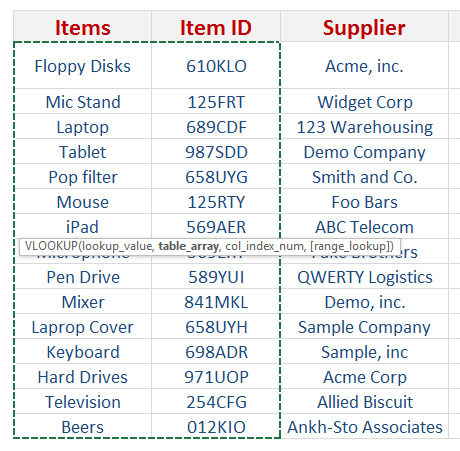STEP 5: You can select the new range with your mouse and also make any changes to the formula bar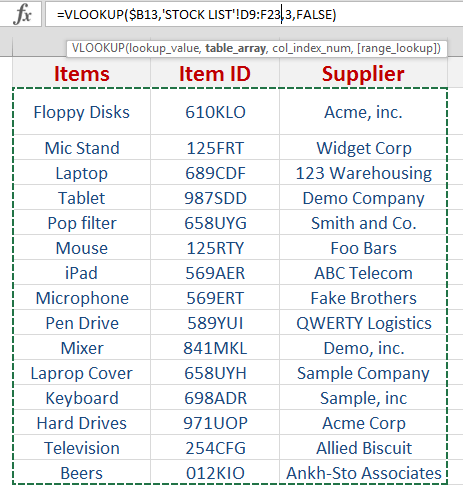STEP 6: Press Enter and your formula is updated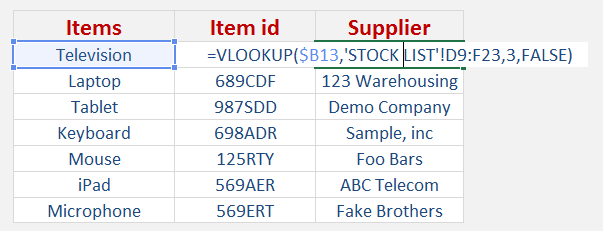## Calculate your Monthly Investment with Excel’s FV Formula

What does it do?

Calculates the compound interest

Formula breakdown:

=FV(rate, nper, pmt, [pv])

What it means:

=FV(interest rate, number of periods, periodic payment, initial amount)

Computing the compound interest of an initial investment is easy for a fixed number of years.  But let’s add an additional challenge.

What if you are also putting in monthly contributions to your investment? Now that’s a lot more challenging to compute now!

How much would be available for you at the end of your investment?

Thankfully there is an easy way to calculate this with Excel’s FV formula! FV stands for Future Value.

In our example below, we have the table of values that we need to get the compound interest or Future Value from: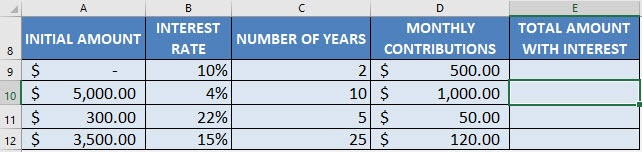There are two important concepts we need to use since we are using monthly contributions:

• Since our interest rate is the annual rate, we will have to divide it by 12 to make it monthly
• We will need to convert our number of years into number of months by multiplying it by 12

I explain how you can do this below:

STEP 1: We need to enter the FV function in a blank cell:

## =FV(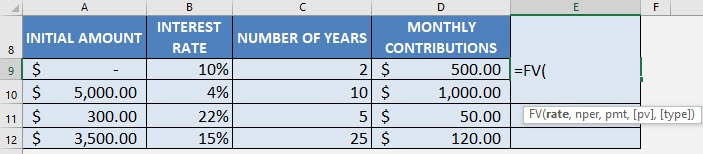STEP 2: The FV arguments:

## rate

What is the rate of the interest?

Select the cell containing the interest rate and divide it by 12 to get the monthly interest rate (make sure that this is in a percentage):

## =FV(B9/12,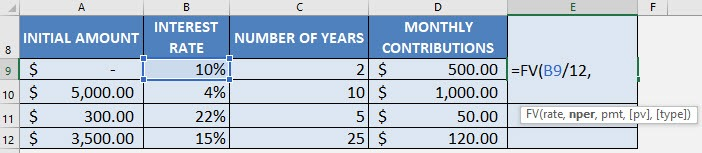## nper

How many periods?

Select the cell containing the number of years and multiply it by 12 to get the number of months:

## =FV(B9/12, C9*12,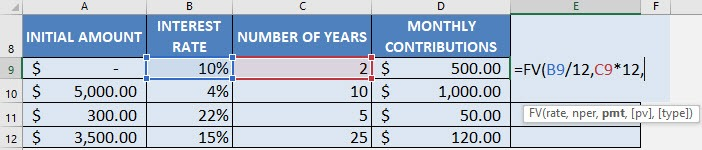## pmt

What is the periodic payment?

Select the cell that contains your monthly contribution (this is your periodic payment):

## =FV(B9/12, C9*12, D9,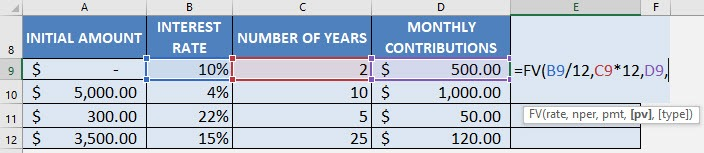## pv

What is the initial amount?

PV stands for present value, the initial amount. Multiply the entire result by -1.

## =FV(B9/12, C9*12, D9, A9) * -1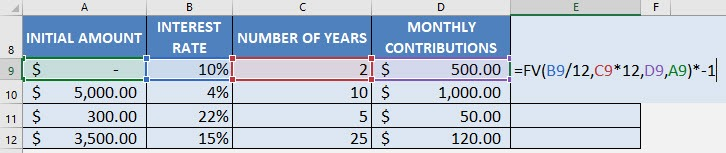Apply the same formula to the rest of the cells by dragging the lower right corner downwards.

You now have all of the compound interest results!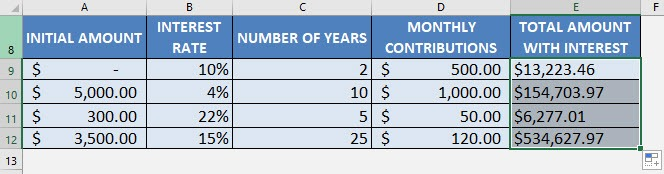## How to Remove Formulas in Excel

There are times when I have an Excel worksheet full of formulas and I want to hard code the results and remove the formulas completely.

This is very easy to do in Excel!

Here is our sample worksheet which has the following formulas in Column E: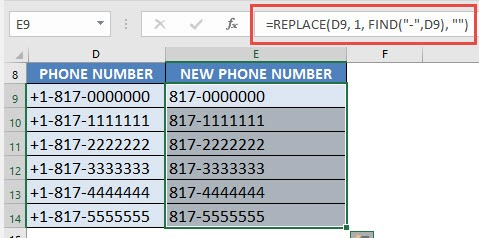I explain how you can remove formulas in Excel below:

STEP 1: Select all the cells that have formulas:STEP 2: Right click and select Copy: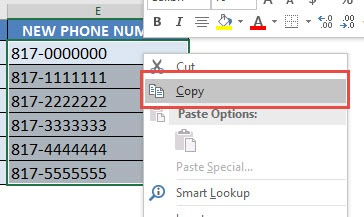STEP 3: Right click again and select Paste Values: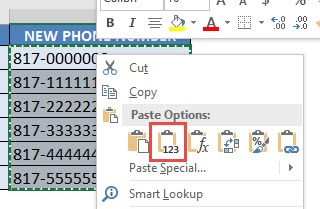Now you will see that the values are only retained and the formulas are now gone!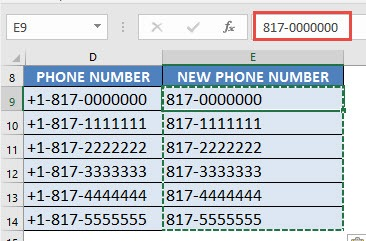Latest Tutorials

March 3, 2021

January 27, 2021

January 18, 2021

## 5 Ways of Using Excel as a Time Value of Money Calculator

September 2, 2020

June 26, 2020

June 26, 2020